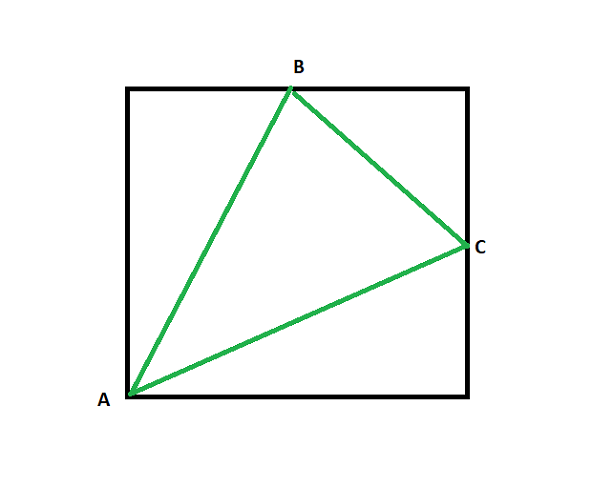GFG App
Open AppBrowser
Continue

# Area of a triangle with two vertices at midpoints of opposite sides of a square and the other vertex lying on vertex of a square

Given a positive integer N representing the side of a square, the task is to find the area of a triangle formed by connecting the midpoints of two adjacent sides and vertex opposite to the two sides.

Examples:

Input: N = 10
Output: 37.5

Input: N = 1
Output: 0.375

Approach: The given problem can be solved based on the following observations:• The one side of the triangle will be the hypotenuse of the triangle formed with the vertices as two middle point and one vertex of the square at the intersection of the sides whose length of the side is given by.
• The length of the other two sides of the triangle is given by.
• Now, the sides of the triangle are known, therefore, the area of the triangle can be calculated using the Heron’s Formula.

Follow the steps below to solve the problem:

Below is the implementation of the above approach:

## C++

 // C++ program for the above approach   #include  using namespace std;   // Function to find the area of the // triangle that inscribed in square double areaOftriangle(int side) {     // Stores the length of the first     // side of triangle     double a = sqrt(pow(side / 2, 2)                     + pow(side / 2, 2));       // Stores the length of the second     // side of triangle     double b = sqrt(pow(side, 2)                     + pow(side / 2, 2));       // Stores the length of the third     // side of triangle     double c = sqrt(pow(side, 2)                     + pow(side / 2, 2));       double s = (a + b + c) / 2;       // Stores the area of the triangle     double area = sqrt(s * (s - a)                        * (s - b) * (s - c));       // Return the resultant area     return area; }   // Driver Code int main() {     int N = 10;     cout << areaOftriangle(N);       return 0; }

## Java

 // Java program for the above approach import java.util.*;   class GFG{    // Function to find the area of the // triangle that inscribed in square static double areaOftriangle(int side) {           // Stores the length of the first     // side of triangle     double a = Math.sqrt(Math.pow(side / 2, 2) +                           Math.pow(side / 2, 2));       // Stores the length of the second     // side of triangle     double b = Math.sqrt(Math.pow(side, 2) +                           Math.pow(side / 2, 2));       // Stores the length of the third     // side of triangle     double c = Math.sqrt(Math.pow(side, 2) +                           Math.pow(side / 2, 2));       double s = (a + b + c) / 2;       // Stores the area of the triangle     double area = Math.sqrt(s * (s - a) *                             (s - b) * (s - c));       // Return the resultant area     return area; }    // Driver code public static void main(String[] args) {     int N = 10;           System.out.print(areaOftriangle(N)); } }   // This code is contributed by sanjoy_62

## Python3

 # Python3 program for the above approach from math import sqrt   # Function to find the area of the # triangle that inscribed in square def areaOftriangle(side):           # Stores the length of the first     # side of triangle     a = sqrt(pow(side / 2, 2) + pow(side / 2, 2))       # Stores the length of the second     # side of triangle     b = sqrt(pow(side, 2) + pow(side / 2, 2))       # Stores the length of the third     # side of triangle     c = sqrt(pow(side, 2) + pow(side / 2, 2))       s = (a + b + c) / 2       # Stores the area of the triangle     area = sqrt(s * (s - a) * (s - b) * (s - c))       # Return the resultant area     return round(area, 1)   # Driver Code if __name__ == '__main__':           N = 10           print (areaOftriangle(N))   # This code is contributed by mohit kumar 29

## C#

 // C# program for the above approach using System; class GFG{    // Function to find the area of the // triangle that inscribed in square static double areaOftriangle(int side) {           // Stores the length of the first     // side of triangle     double a = Math.Sqrt(Math.Pow(side / 2, 2) +                           Math.Pow(side / 2, 2));       // Stores the length of the second     // side of triangle     double b = Math.Sqrt(Math.Pow(side, 2) +                           Math.Pow(side / 2, 2));       // Stores the length of the third     // side of triangle     double c = Math.Sqrt(Math.Pow(side, 2) +                           Math.Pow(side / 2, 2));       double s = (a + b + c) / 2;       // Stores the area of the triangle     double area = Math.Sqrt(s * (s - a) *                             (s - b) * (s - c));       // Return the resultant area     return area; }    // Driver code public static void Main(string[] args) {     int N = 10;           Console.WriteLine(areaOftriangle(N)); }}   // This code is contributed by ukasp.

## Javascript

 

Output:

37.5

Time Complexity: O(logn) because using inbuilt sqrt function
Auxiliary Space: O(1)

My Personal Notes arrow_drop_up